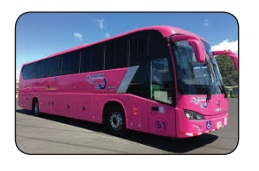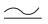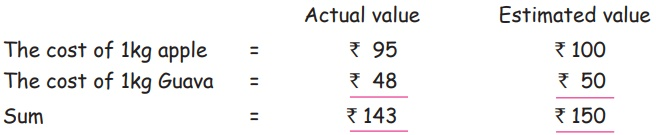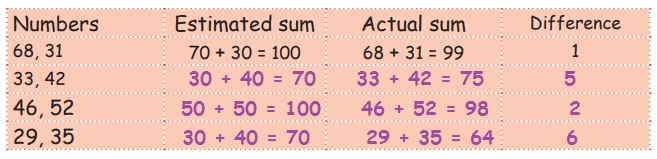Home | | Maths 5th Std | Estimation

# Estimation

Let us Know: Other words denoting estimates are 'About', 'Approximate', 'nearby', ……….

UNIT−2

NUMBERSEstimation

Situation 1

There are two bunches of grapes find the number of grapes in bunch ‘A’ and bunch ‘B’ without counting it.Number of grapes in bunch ‘A’ 110

Number of grapes in bunch ‘B’ 60

Then count the exact number of grapes and note it.

Exact number of grapes in bunch ‘A’ 118

Exact number of grapes in bunch ‘B’ 64

Situation 2

The estimated bus fare for a trip from trichy to Chennai is 300. The Exact bus fare is 286Therefore, the value which is nearest to the correct value is called its estimated value.

The symbol for estimate isLet us Know: Other words denoting estimates are 'About', 'Approximate', 'nearby', ……….

Activity 1

Fill and enjoy (individual)Able to estimate a number upto two digit

Estimating a number to our desired number is called estimation.

To estimate a number, first underline the digit that is to be estimated. If the underlined digit is less than 5 then the number should be rounded down to the nearest underlined digit.

For example, when we estimate 64 to its nearest Tens place the number have to be rounded down to 60.

If the underlined digit is greater than or equal to 5, then the number should be rounded upto the nearest underlined digit.

For example, when we estimate 65 to its nearest Tens place the number have to be rounded upto 70.

Example 2.1

Estimate 48 to its nearest Tens place.

Solution

Estimating 48 to its nearest Tens place, we get 50.

Since the unit place is 8, which is greater than 5, the number have to be rounded upto 50.

Therefore, 48 50.

Example 2.2

Estimate 74 to its nearest Tens place.

Solution

Estimating 74 to its nearest Tens place, we get 70.

Since the unit place is 4, which is less than 5, the number have to be rounded down to 70.

Therefore, 74 70.

Example 2.3

Estimate 144 to its nearest Tens place.

Solution

Estimating 144 to its nearest Tens place, we get 140.

Since the unit place is 4, which is less than 5, the number have to be rounded down to 140.

Therefore, 144 140.

Example 2.4

Estimate 155 to its nearest Tens place.

Solution

Estimating 155 to its nearest Tens place, we get 160.

Since the unit place is 5, which is equal to 5, the number have to be rounded upto 160.

Therefore, 155 160.

Able to estimate sums, differences products and quotients up to two digit numbers.

Example 2.5

If the cost of 1 kg apple is ₹ 95 and the cost of 1 kg Guava is ₹ 48 then estimate their cost nearest to Tens place and find their sum. Also, find the difference between the estimated sum and actual sum.

SolutionDifference between estimated value and actual value  = Estimated value – Actual value

= 150 − 143

= ₹ 7

Example 2.6

If the cost of a note book is ₹ 42 and the cost of a pen is ₹ 27, then estimate their cost nearest to Tens place and find their difference. Also, find the difference between the estimated value and actual value.

SolutionDifference between estimated value and actual value  = Actual value – Estimated value

= 15−10

= ₹ 5

Try this

Write down the estimated value of numbers and find their sum. Also, find the difference of their sum.Example 2.7

If the cost of 1 metre cloth is ₹ 86, then estimate its cost nearest to Tens place and find the cost of 5 metre cloth.

SolutionTherefore, the cost of 5 metre cloth is ₹ 450

Example 2.8

If the cost of 3 kg potato is ₹ 63, then estimate its cost nearest to Tens place and find the cost of 1 kg potato.

SolutionTherefore, the cost of 1 kg potato is ₹20

Try this

Write down the estimated value of numbers and divide. Then find the difference between estimated value and actual value.Tags : Numbers | Term 3 Chapter 2 | 5th Maths , 5th Maths : Term 3 Unit 2 : Numbers
Study Material, Lecturing Notes, Assignment, Reference, Wiki description explanation, brief detail
5th Maths : Term 3 Unit 2 : Numbers : Estimation | Numbers | Term 3 Chapter 2 | 5th Maths